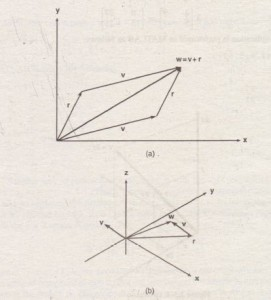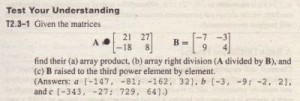2.3  Element-by-Element Operations

To increase the magnitude of a vector, multiply it by a scalar. For example, to double the magnitude of the vector r = [ 3 , 5, 2] •multiply each component by two to obtain [6, 10 , 4 ] . In MATLAB you type v = 2 *r. See Figure 2.3-1 for the geometric interpretation of scalar multiplication of a vector in three dimensional space. Multiplying a matrix A by a scalar w produces a matrix whose elements are the elements of A multiplied hf w. For exampleFigure 2.3-1 Geometric
interpretation of scalar
multiplication of a vector.

Thus multiplication of an array by a scalar is easily defined and easily carried out. However, multiplication of two arrays is not so straightforward. In fact. MATLAB uses two definitions of multiplication: (l) array multiplication and
(2) matrix multiplication. Division and exponentiation must also be carefully defined when you are dealing with operations between two arrays. MATLAB has two forms of arithmetic operations on arrays. In this section we introduce one form, called array operations, which are also called element-by-element operations. In the next section we introduce matrix-operations. Each form has its own applications, which we illustrate by examples.

Vector addition can be done either graphically (by using the parallelogram law in two dimensions (see Figure 2.3-2a), or analytically by adding the correspondingFigure 2.3-2 ‘yector addition. (a) The parallelogram law.
(b) Addition of vectors in three dimensions.

components. To add the vectors r = [ 3 , 5 , 2) and v = [ 2 , – 3 , 1) to create w in MATLAB, you type w = r + v. The result is w = [5, 2 , 3). Figure 2.3-2b illustrates vector addition in three dimensions. When two arrays have identical size, their sum or difference has the same size and is obtained by adding or subtracting their corresponding elements. Thus C = A + B implies that cij = aij + bij if the arrays are matrices. The array C has the same size as A nd B. For example:

Array subtraction is performed in a, similar way. •. . ‘.”l: The addition shown in equation 2.3-1 is performed in MATLAB asfollows:

»A = [6, -2; 10,3) ;

»B = [9,8;-12,14)

A+B
ans =
15 6
-2 17
Array addition and subtraction are associative and commutative. For addition these properties mean that

( A +  B ) + C = A + ( B + C )             (2.3-2)
A + B + C = B + C + A = A + c + B          (2.3-3)

Array addition and subtraction require that both arrays have the same size. The only exception to this rule in MATLAB occurs when we add or subtract a scalar to or from an array. In this case the scalar is added or subtracted from each element in the array. Table 2.3-1 gives examples.Table 2.3-1 Element-by-element operations

Array Multiplication
The data in Table 2.3-2 illustrates the difference between the two types of multiplication that are defined in ~TLAB. The table gives the speed of an aircraft on each leg of a certain trip and the time spent on each leg. We can define a row vector’ s containing the speeds and: a row vector t containing the times for each leg. Thus s = [ 2 00 I 250 I 400 I 300] and t = [ 2 I 5 I 3 I 4 ] . To find the miles traveled on each leg, we multiply the speed by the time. To do so, we use the MATLAB symbol . *, which specifies the multiplication s . * t to produce the row vector whose elements are the products of the corresponding elements in s and t:

s. *:t·=[200(2),250(51,400(3 ,300(4)] = [400, 1250, 1200, 1200]

With this notation the symbol .. * sigmfies that each element in s is multiplied by
the corresponding element in t and that the resulting products are used to form a row vector having the same number of elements as s and t. This vector contains the miles traveled by the aircraft on each leg of the trip. .
If we had wanted to find.only tile total miles traveled, we could have used another definition of multiplication, denoted by s*t I • In this definition the productis the sum of the individual element products; that is

s*t I = [200(2)·+ 250(5) + 400(3) + 300(4)] =4050

These two examples illustrate the difference between array multiplication s . * t-sometimes called element-by-element multiplication-and matrix multiplication s * t I. We examine matrix multiplication in more detail in
Sectioo2.4.

MATLAB defines element-by-element multiplication only for arrays that have the same size. The definition of the product x . *y, where x and y each have n elements, is

x .-*y = (x (1 ) y (1) I X ( 2 )y (2) I ••• I X (n )y (n ) 1Note that x I is a column vector with size 3 x 1 and thus does not have the same size as y, whose size is 1 x 3. Thus for the vectors x and y the operations x I • *y and y. *x I are not defined in MATLAB and will generate an error
message.
The generalization of array multiplication to arrays with more than one row or column is straightforward. Both arrays must have the same size. If they are matrices, they must have the same number of rows and the same number of columns.The array operations are performed between the elements in corresponding locations
in the arrays. For example, the array multiplication operation A. *B results in a matrix C that has the same size as A and B and has the elements cij = aijbij For example, ifWith element-by-element multiplication, it is important to remember that the dot (.) and the asterisk (*) form one symbol It might have been better to bave defined a single symbol for this operation, but the developers of MATLAB were limited by the selection of symbols on the keyboard .

The built-in MArtAB functions such as sqrt (x ) and exp (x ) automatically operate on array arguments to produce an array result the same size as the array argument x. Thus these functions are said to be vectorized functions.
For example, in the following session the. result y has the same size as the argument x.

»x = [4, 16, 25] ;
»y = sqrt(xl
Y=
2 4 5

However, when multiplying or dividing these functions, or wren raising them to a power, you must use element-by-element operations if the arguments are arrays. For example, to compute z = (eY sin x)cos2 x, you must Itype Z = exp (y) . *sin (x ) . * (cos (x) ) . “2. Obviously, you will get an ,rror message if the size of x is not the same as the size of y. The result z will have the same size as x and y.

Array Division
The definition of array division, also called element-by-element division, is similar to the definition of array multiplication except, of course, that the elements of one array are divided by the elements of the other array. Both arrays must have the same size. The symbol for array right division is . I. For example, ifThe array left division operator (. \) is defined to perform element-by-element division using left division. Refer to Table 2.3-1 for examples. Note that A. \B is not equivalent to A. lB.

Array Exponentiation
MATLAB enables us not only to raise arrays to powers but also to raise scalars I , and arrays to array powers. To perform exponentiation.on an element-by-element basis, we must use the . “, symbol. For example, ‘if x = [ 3 I 5 , 8], then typingx.”‘ 3 produces the array [33, 53, 83]=[27,125, 512].Ifx = [0:2:6],We can raise a scalar to an array power. For example, if p = [ 2, 4, 5]. then typing 3 . “‘p produces the array [32, 34, 35] = [9, 81, 243]. This example illustrates a common situation in which it helps to remember that . ‘” is a single
‘symbol; the dot in 3 . “‘p is not a decimal point associated with the number 3. The following operations, with the value of p given here, are equivalent and givethe correct answer:

3.”‘p
3.0.”‘p
3 .. “‘p
(3) • “‘p
3.”‘[2,4,5]USing Array Operations to~valuate MultivariableFunctions
To evaluate a function oftwo variables, say, z = f(x, Y), using array operations, for the values x = Xl~ X2, •…. , Xm and y = YJ, Y2, , Ym, define the m x n matrices:When the function z = f tx , y) is evaluated in MATLAB using array operations, the-resulting 111 x 11 matrix z has the elements Z;j = I(x;, Yj). We can extend this technique to functions of more than two variables by using multidimensional arrays